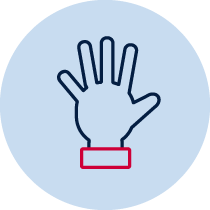# Addition and subtraction – What's the difference?

Students use and record a range of mental strategies to solve addition and subtraction problems involving two-digit numbers and explain or demonstrate how an answer was obtained for addition and subtraction problemsPractical Resource required Verbal recording Take photo Teacher observation

## Number and algebra – addition and subtraction 2

• supports conclusions by explaining or demonstrating how answers were obtained MA1-3WM
• uses a range of strategies and informal recording methods for addition and subtraction involving one- and two-digit numbers MA1-5NA

## Syllabus content descriptors

Solve simple addition and subtraction problems using a range of efficient mental and written strategies (ACMNA030)

## Linked syllabus outcome and content descriptors

Patterns and algebra 2

• use equivalent number sentences involving addition and subtraction to find unknown quantities (ACMNA083)

## Materials

• Numeral cards 0-9 or playing cards A=1 to 10
• Paper, pencil, whiteboard, device for recording

## Teacher instructions

This task is designed to determine student understanding of the concepts:

• use and record a range of mental strategies to solve addition and subtraction problems involving two-digit numbers, including the jump strategy on an empty number line
• explain or demonstrate how an answer was obtained for addition and subtraction problems

Provide each student with paper, a whiteboard or a device for recording and a set of numeral or playing cards in the range 0–9. Have the students draw two cards from the pile and construct the lowest two-digit number possible from the combination. Record the numeral on their paper, whiteboard or device.

The student then reverses the numerals and records the largest number that can be made. Have the students determine the difference between the two numbers and record this number and share their strategies. If the same number is drawn for both cards, have the students return one to the pile and redraw another card.

Enabling questions:

• What do you know, what do you need to find out?

## Student instructions

You need to take turns to draw two cards from the pile. Make and record the smallest 2-digit number that you can (what you have).

Now make the two cards into the largest number that you can (what you need). Record this on your paper, whiteboard or device.

You need to find the number that is missing or the difference between the smallest and the largest number (what I want). Record this on your paper, whiteboard or device.

You need to share what strategies you used.

## Where to next?

• supply concrete materials such as Unifix or MAB resources to make and manipulate the numbers
• explicitly teach students how to write a number sentence including the numbers and symbols that are known (see Stage 1 diagnostic task ‘What numbers are missing?’)
• explicitly teach how to record their thinking using an empty number line to solve addition and subtraction

Students:

• could solve the task using 2-digit numbers - ask students to complete the task using 3 cards to create 3-digit numbers to find the difference or one 3-digit number and a 2-digit number
• create different combinations using cards to make two numbers to add or subtract and they have to find the answer

Adapted from Developing Efficient Numeracy Strategies DENS 2

Connects to Stage 1 diagnostic task ‘What numbers are missing?’ and Stage 2 task p.8